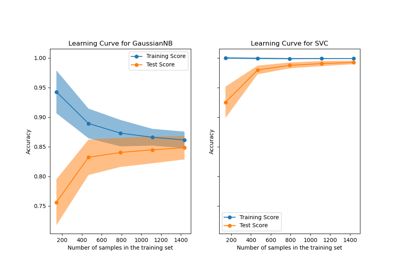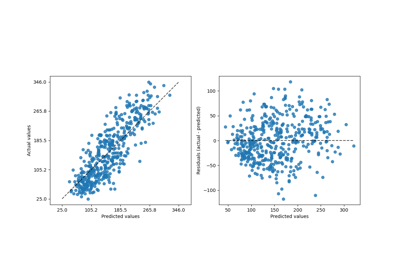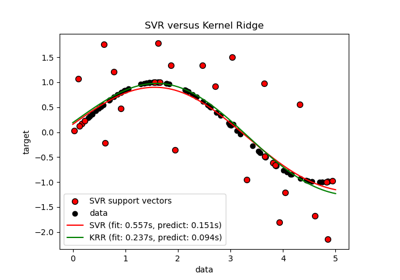# sklearn.model_selection.LearningCurveDisplay¶

class sklearn.model_selection.LearningCurveDisplay(*, train_sizes, train_scores, test_scores, score_name=None)[source]

Learning Curve visualization.

It is recommended to use from_estimator to create a LearningCurveDisplay instance. All parameters are stored as attributes.

Read more in the User Guide for general information about the visualization API and detailed documentation regarding the learning curve visualization.

New in version 1.2.

Parameters:
train_sizesndarray of shape (n_unique_ticks,)

Numbers of training examples that has been used to generate the learning curve.

train_scoresndarray of shape (n_ticks, n_cv_folds)

Scores on training sets.

test_scoresndarray of shape (n_ticks, n_cv_folds)

Scores on test set.

score_namestr, default=None

The name of the score used in learning_curve. It will override the name inferred from the scoring parameter. If score is None, we use "Score" if negate_score is False and "Negative score" otherwise. If scoring is a string or a callable, we infer the name. We replace _ by spaces and capitalize the first letter. We remove neg_ and replace it by "Negative" if negate_score is False or just remove it otherwise.

Attributes:
ax_matplotlib Axes

Axes with the learning curve.

figure_matplotlib Figure

Figure containing the learning curve.

errorbar_list of matplotlib Artist or None

When the std_display_style is "errorbar", this is a list of matplotlib.container.ErrorbarContainer objects. If another style is used, errorbar_ is None.

lines_list of matplotlib Artist or None

When the std_display_style is "fill_between", this is a list of matplotlib.lines.Line2D objects corresponding to the mean train and test scores. If another style is used, line_ is None.

fill_between_list of matplotlib Artist or None

When the std_display_style is "fill_between", this is a list of matplotlib.collections.PolyCollection objects. If another style is used, fill_between_ is None.

sklearn.model_selection.learning_curve

Compute the learning curve.

Examples

>>> import matplotlib.pyplot as plt
>>> from sklearn.model_selection import LearningCurveDisplay, learning_curve
>>> from sklearn.tree import DecisionTreeClassifier
>>> tree = DecisionTreeClassifier(random_state=0)
>>> train_sizes, train_scores, test_scores = learning_curve(
...     tree, X, y)
>>> display = LearningCurveDisplay(train_sizes=train_sizes,
...     train_scores=train_scores, test_scores=test_scores, score_name="Score")
>>> display.plot()
<...>
>>> plt.show()


Methods

 from_estimator(estimator, X, y, *[, groups, ...]) Create a learning curve display from an estimator. plot([ax, negate_score, score_name, ...]) Plot visualization.
classmethod from_estimator(estimator, X, y, *, groups=None, train_sizes=array([0.1, 0.33, 0.55, 0.78, 1.]), cv=None, scoring=None, exploit_incremental_learning=False, n_jobs=None, pre_dispatch='all', verbose=0, shuffle=False, random_state=None, error_score=nan, fit_params=None, ax=None, negate_score=False, score_name=None, score_type='both', log_scale='deprecated', std_display_style='fill_between', line_kw=None, fill_between_kw=None, errorbar_kw=None)[source]

Create a learning curve display from an estimator.

Read more in the User Guide for general information about the visualization API and detailed documentation regarding the learning curve visualization.

Parameters:
estimatorobject type that implements the “fit” and “predict” methods

An object of that type which is cloned for each validation.

Xarray-like of shape (n_samples, n_features)

Training data, where n_samples is the number of samples and n_features is the number of features.

yarray-like of shape (n_samples,) or (n_samples, n_outputs) or None

Target relative to X for classification or regression; None for unsupervised learning.

groupsarray-like of shape (n_samples,), default=None

Group labels for the samples used while splitting the dataset into train/test set. Only used in conjunction with a “Group” cv instance (e.g., GroupKFold).

train_sizesarray-like of shape (n_ticks,), default=np.linspace(0.1, 1.0, 5)

Relative or absolute numbers of training examples that will be used to generate the learning curve. If the dtype is float, it is regarded as a fraction of the maximum size of the training set (that is determined by the selected validation method), i.e. it has to be within (0, 1]. Otherwise it is interpreted as absolute sizes of the training sets. Note that for classification the number of samples usually have to be big enough to contain at least one sample from each class.

cvint, cross-validation generator or an iterable, default=None

Determines the cross-validation splitting strategy. Possible inputs for cv are:

• None, to use the default 5-fold cross validation,

• int, to specify the number of folds in a (Stratified)KFold,

• An iterable yielding (train, test) splits as arrays of indices.

For int/None inputs, if the estimator is a classifier and y is either binary or multiclass, StratifiedKFold is used. In all other cases, KFold is used. These splitters are instantiated with shuffle=False so the splits will be the same across calls.

Refer User Guide for the various cross-validation strategies that can be used here.

scoringstr or callable, default=None

A string (see The scoring parameter: defining model evaluation rules) or a scorer callable object / function with signature scorer(estimator, X, y) (see Defining your scoring strategy from metric functions).

exploit_incremental_learningbool, default=False

If the estimator supports incremental learning, this will be used to speed up fitting for different training set sizes.

n_jobsint, default=None

Number of jobs to run in parallel. Training the estimator and computing the score are parallelized over the different training and test sets. None means 1 unless in a joblib.parallel_backend context. -1 means using all processors. See Glossary for more details.

pre_dispatchint or str, default=’all’

Number of predispatched jobs for parallel execution (default is all). The option can reduce the allocated memory. The str can be an expression like ‘2*n_jobs’.

verboseint, default=0

Controls the verbosity: the higher, the more messages.

shufflebool, default=False

Whether to shuffle training data before taking prefixes of it based ontrain_sizes.

random_stateint, RandomState instance or None, default=None

Used when shuffle is True. Pass an int for reproducible output across multiple function calls. See Glossary.

error_score‘raise’ or numeric, default=np.nan

Value to assign to the score if an error occurs in estimator fitting. If set to ‘raise’, the error is raised. If a numeric value is given, FitFailedWarning is raised.

fit_paramsdict, default=None

Parameters to pass to the fit method of the estimator.

axmatplotlib Axes, default=None

Axes object to plot on. If None, a new figure and axes is created.

negate_scorebool, default=False

Whether or not to negate the scores obtained through learning_curve. This is particularly useful when using the error denoted by neg_* in scikit-learn.

score_namestr, default=None

The name of the score used to decorate the y-axis of the plot. It will override the name inferred from the scoring parameter. If score is None, we use "Score" if negate_score is False and "Negative score" otherwise. If scoring is a string or a callable, we infer the name. We replace _ by spaces and capitalize the first letter. We remove neg_ and replace it by "Negative" if negate_score is False or just remove it otherwise.

score_type{“test”, “train”, “both”}, default=”both”

The type of score to plot. Can be one of "test", "train", or "both".

log_scalebool, default=”deprecated”

Whether or not to use a logarithmic scale for the x-axis.

Deprecated since version 1.3: log_scale is deprecated in 1.3 and will be removed in 1.5. Use display.ax_.xscale and display.ax_.yscale instead.

std_display_style{“errorbar”, “fill_between”} or None, default=”fill_between”

The style used to display the score standard deviation around the mean score. If None, no representation of the standard deviation is displayed.

line_kwdict, default=None

Additional keyword arguments passed to the plt.plot used to draw the mean score.

fill_between_kwdict, default=None

Additional keyword arguments passed to the plt.fill_between used to draw the score standard deviation.

errorbar_kwdict, default=None

Additional keyword arguments passed to the plt.errorbar used to draw mean score and standard deviation score.

Returns:
displayLearningCurveDisplay

Object that stores computed values.

Examples

>>> import matplotlib.pyplot as plt
>>> from sklearn.model_selection import LearningCurveDisplay
>>> from sklearn.tree import DecisionTreeClassifier
>>> tree = DecisionTreeClassifier(random_state=0)
>>> LearningCurveDisplay.from_estimator(tree, X, y)
<...>
>>> plt.show()

plot(ax=None, *, negate_score=False, score_name=None, score_type='both', log_scale='deprecated', std_display_style='fill_between', line_kw=None, fill_between_kw=None, errorbar_kw=None)[source]

Plot visualization.

Parameters:
axmatplotlib Axes, default=None

Axes object to plot on. If None, a new figure and axes is created.

negate_scorebool, default=False

Whether or not to negate the scores obtained through learning_curve. This is particularly useful when using the error denoted by neg_* in scikit-learn.

score_namestr, default=None

The name of the score used to decorate the y-axis of the plot. It will override the name inferred from the scoring parameter. If score is None, we use "Score" if negate_score is False and "Negative score" otherwise. If scoring is a string or a callable, we infer the name. We replace _ by spaces and capitalize the first letter. We remove neg_ and replace it by "Negative" if negate_score is False or just remove it otherwise.

score_type{“test”, “train”, “both”}, default=”both”

The type of score to plot. Can be one of "test", "train", or "both".

log_scalebool, default=”deprecated”

Whether or not to use a logarithmic scale for the x-axis.

Deprecated since version 1.3: log_scale is deprecated in 1.3 and will be removed in 1.5. Use display.ax_.set_xscale and display.ax_.set_yscale instead.

std_display_style{“errorbar”, “fill_between”} or None, default=”fill_between”

The style used to display the score standard deviation around the mean score. If None, no standard deviation representation is displayed.

line_kwdict, default=None

Additional keyword arguments passed to the plt.plot used to draw the mean score.

fill_between_kwdict, default=None

Additional keyword arguments passed to the plt.fill_between used to draw the score standard deviation.

errorbar_kwdict, default=None

Additional keyword arguments passed to the plt.errorbar used to draw mean score and standard deviation score.

Returns:
displayLearningCurveDisplay

Object that stores computed values.

## Examples using sklearn.model_selection.LearningCurveDisplay¶Plotting Learning Curves and Checking Models’ Scalability

Plotting Learning Curves and Checking Models' Scalability

## Examples using sklearn.model_selection.LearningCurveDisplay.from_estimator¶Release Highlights for scikit-learn 1.2

Release Highlights for scikit-learn 1.2Comparison of kernel ridge regression and SVR

Comparison of kernel ridge regression and SVRPlotting Learning Curves and Checking Models’ Scalability

Plotting Learning Curves and Checking Models' Scalability